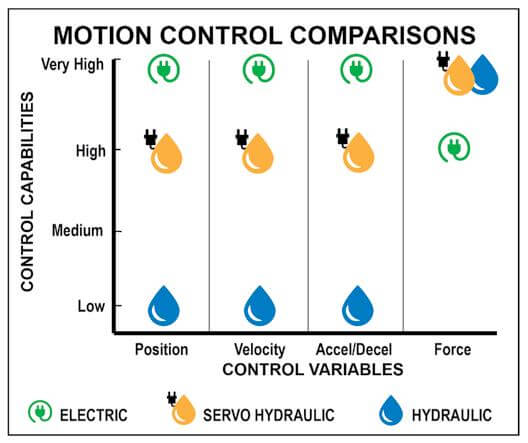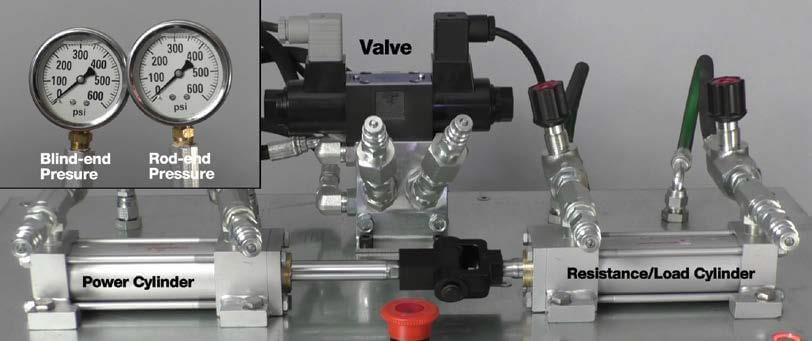# High force linear actuators: how to convert from hydraulic to electric

By Ryan Klemetson on January 22, 2019Electric linear actuators have come a long way – especially in the area of high force.  Once upon a time when an application required high force, the usual linear motion solution was a hydraulic cylinder. However, as industrial automation gets more sophisticated and the need for precise control of speed, force and other variables grows, more engineers are considering electric high force linear actuators.

Now, it often happens that new high-force linear actuator applications go with electric actuators. Also, machine designers are converting existing linear motion systems from hydraulic to electric due to the technology’s many benefits. (Read about hydraulic cylinders versus electric roller screw actuators here.)

When converting a new or existing application to electric actuators, the process involves considering the actual force output of the cylinder, the duty cycle and the motion profile.  Our recommended best practices for conversion are in our new Guide, How to convert hydraulic cylinders to an electric actuator alternative. Download your copy here.

## Ways to figure the force

There are various ways to determine the actual force output of a hydraulic cylinder in an application.  Since these components operate using pressurized oil inside the cylinder bore, you can use the basic formula: Force = Area x Pressure. A simple calculation based on the cylinder’s bore and the rated system pressure will provide an estimate of the potential force output.

However, this calculation may not give you the true story. The Force = Area x Pressure calculation will provide the force the cylinder could optimally deliver. Since it’s common practice to over-size cylinders, using this simple calculation will overstate the force required. It’s much more accurate to use the application’s actual values, as described here.

Let’s look at a real-life example: an application with a 3.5” (100mm) diameter cylinder with a 1.5” (45mm) diameter rod operating with a servo-hydraulic valve. During the cylinder’s extend stroke, the maximum pressure is 1,500 PSI (103 Bar). On the rod end of the cylinder, the down-side (or return line to the reservoir) pressure is 1,000 PSI (69 Bar).

Here are two common ways that output force might be calculated:

OPTION 1 – System Pressure and Piston Area Only:

Force = Area (π x r2) x Pressure

Force = (π x 1.752) x 2,500

Force = 9.62 x 2,500

Force = 24,050 lbf (107 kN)

OPTION 2 – Work Port Pressure and Piston Area Only:

Force = Area (π x r2) x Pressure

Force = (π x 1.752) x 1,500

Force = 9.62 x 1,500

Force = 14,430 lbf (64.2 kN)

## Recommended best practices

Neither of these methods is very precise, and precision matters with electric linear actuators. While the cost of an oversized hydraulic cylinder may not hurt the budget, an oversized electric actuator will be expensive and harm the project’s return on investment.

To get a precise calculation of output force, we recommend a method which looks at the difference between input and output force.

Force = (A1 x P1) – (A2 x P2)

A1 = Piston surface area

A2 = Effective surface area of cylinder

Area = π x r2

P1 = Blind-end (piston side) pressure (PSI) or (Bar)

P2 = Rod-end pressure (PSI) or (Bar)

Using this formula as a third option, we have:

Force = (A1 x P1) – (A2 x P2)

Force = [(π x 1.752) x 1,500] – {[(π x 1.752) – (π x 1.752)] x 1,000}

Force = (9.62 x 1,500) – (7.85 x 1,000)

Force = 14,430 – 7,850

Force = 6,580 lbf (29.28 kN)

Watch our video on this method.There’s a 70% difference between this result [6,580 lbf (29.28 kN)] and that of Option 1 [24,050 lbf (107 kN)].  That translates into a major cost difference when considering an electric actuator. Imagine the potential for savings.

## In summary

It makes sense to convert many hydraulic linear motion applications to electric. Electric high-force linear actuators offer improved control of process variables, better accuracy, the ability to handle complex motion profiles and many other benefits. But appropriately sizing an electric actuator is crucial because an oversized electric actuator will add unnecessary cost to a project.

How you measure the force of a hydraulic cylinder affects your ability to select an appropriate electric high-force linear actuator.   To get to thrust force measurements that allow you to select the best electric linear actuator, measure the working pressures as close as possible to the hydraulic cylinder ports and on both sides of the cylinder.

## What’s next?

The next issue of our blog will look at describing the application’s motion profile and the other remaining pieces of information you need when converting an application from hydraulic to electric actuation.

## Resources

For our recommended best practices for conversion, download our new Guide, How to convert hydraulic cylinders to an electric actuator alternative.